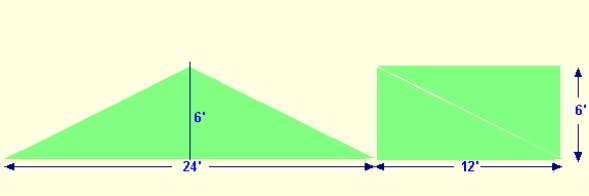Spike's Calculators

# Calculate the Square Footage of a Gable

To calculate the square footage of a gable wall section, you need to know the wall length and the height of the gable. If for some reason you can't get the height of the gable, you don't have a ladder long enough, or you are one of those that should not be at heights if you know the pitch of the roof and the wall length, you can also calculate the square footage of a gable.### Calculating gable height, knowing wall length and roof pitch;

This calculation is done using the same units. Using mixed units, like feet and inches, can make it hard to do the calculation.

```gable wall-length = 24 feet ( 12 * 24 = 288 inches)
roof pitch = 6 : 12 a 6-inch rise for every 12 inches of run```

Take half the length of the gable wall and divide this by the run of 12 inches;

`288 ÷ 2 = 144`
`Half the gable wall has a length of 144 inches.`

Measured from outside to the centre of the gable, at 144 inches, is the location of the peak. At this location is the highest point of the gable.

Calculate the number of 12-inch runs that there are in 144 inches;

`144 ÷ 12 = 12`
`There are 12 x 12-inch runs in 144 inches of wall length.`

Each of these run sections increases the height of the roof by 6 inches.

`12 * 6 = 72`
`The gable at the centre has a height of 72 inches = 6 feet.`

##### Calculating the gable's square footage;
```gable wall-length = 24 feet
wall height = 6 feet
```The easiest way to visualize this is to split the gable length into two triangles and form a rectangle.
This rectangle has a length of 12 feet and a height of 6 feet. To calculate the area of the gable, multiply the length by the height measurement;

`6 * 12 = 72`
`The total area of the gable is 72 square feet.`

And one more because it is so much fun!
```gable wall-length = 34 feet 3 inches
roof pitch = 5 : 12 a 5-inch rise for every 12 inches of run```

Get rid of the mixed units used for wall length;

`34 * 12 (12 inches in one foot) = 408 + 3 = 411`
`34 feet 3 inches = 411 inches`

Take half the length of the gable wall and divide this by the run of 12 inches;
`411 ÷ 2 = 205.5`
`Half the length of the gable is 205.5 inches.`

Calculate the number of 12-inch runs that there are in 205.5 inches;
`205.5 ÷ 12 = 17.125`
`There are 17.125 x 12-inch runs in 205.5 inches of wall length.`

Each of these run sections increases the height of the roof by 5 inches.
`17.125 * 5 = 85.625`
`The gable at the centre has a height of 85.625 inches = 7 feet 1 5/8 inches.`

##### Calculating the gable's square footage;
```gable wall-length = 34 feet 3 inches (411 inches)
wall height = 7 feet 1 5/8 ( 85.625 inches)
```

Again, divide the gable wall length in two, creating two triangles. Join the two triangles to form a rectangle.

This rectangle shape has a length of 205.5 inches and a height of 85.625 inches. Multiply the length by the height;

`205.5 * 85.625 = 17595.9375 rounded to 17595`
`This gable has an area of 17595 square inches.`

Convert this to square feet by dividing 17595 inches by 144, ( there are 144 square inches in one square foot);
`17595 ÷ 144 = 122.1875 rounded to 123`
`The area of this gable is 123 square feet.`

If you are doing a rough estimate, you can round the wall length up to 35 feet, multiply this number by 7 (the height) and divide the result by 2;
`35 * 7 = 245 ÷ 2 = 122.5 square feet`

Or better yet, round the 7 feet 1 5/8 to 7.5 feet;
`35 * 7.5 = 262.5 ÷ 2 = 131.25 rounded to 132`
`The estimated area of the gable is 132 square feet.`